# Problem of the Day

Mar 23, 2020March 23, 2020

23.14 + 1.02 = ?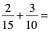Categorize the following Real numbers as : Natural, Whole, Integer, Rational and Irrational. Make sure to include every appropriate category.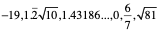Algebra

Add: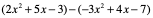Geometry

Describe the side length and angle measurements for equilateral, isosceles and scalene triangles.

Algebra II

Find the vertex and direction of opening for the following parabola.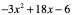Trigonometry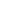You have a right triangle ABC with sides a and b and hypotenuse c. The length of side a is 6. The length of side b is 8. Find the length of side c and set up the 6 trig ratios (sin, cos, tan, csc, sec and cot) for angle A

PreCalculus

Write the transformation of the graph of y = x2  shifted right 4 units, up 2 units and reflected over the x axis.

Grade 7: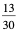Grade 8:  -19 real, rational, integer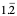real, rational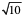real, irrational

1.43186…..   real, irrational

0 real, rational, integer, whole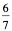real, rational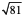real, rational, integer, whole and natural

Algebra: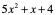Geometry:  equilateral  all sides the same length all angles 60 degrees

isosceles  two sides the same length base angles congruent and measure (180 - vertex angle)/2

scalene  no sides the same length. smallest angle across from shortest side, largest angle across from longest side

Algebra II: x coordinate of the vertex is -b/2a  so -18/-6 or 3

plug into equation to find y coordinate of the vertex = 21

vertex (3, 21) opens down because a<0

Trigonometry: c = 10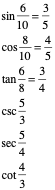Pre-calculus:  -(x-4)2 +2